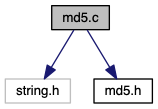networkclient
md5.c File Reference

MD5 functions. More...

`#include <string.h>`
`#include "md5.h"`
Include dependency graph for md5.c:## Macros

#define F(x, y, z)   ((z) ^ ((x) & ((y) ^ (z))))

#define G(x, y, z)   ((y) ^ ((z) & ((x) ^ (y))))

#define H(x, y, z)   (((x) ^ (y)) ^ (z))

#define H2(x, y, z)   ((x) ^ ((y) ^ (z)))

#define I(x, y, z)   ((y) ^ ((x) | ~(z)))

#define STEP(f, a, b, c, d, x, t, s)

#define SET(n)

#define GET(n)   (ctx->block[(n)])

#define OUT(dst, src)

## Functions

void MD5_Init (MD5_CTX *ctx)

void MD5_Update (MD5_CTX *ctx, const void *data, unsigned long size)

void MD5_Final (unsigned char *result, MD5_CTX *ctx)

## Detailed Description

MD5 functions.

This is an OpenSSL-compatible implementation of the RSA Data Security, Inc. MD5 Message-Digest Algorithm (RFC 1321).

Author: Alexander Peslyak, better known as Solar Designer <solar at openwall.com>

This software was written by Alexander Peslyak in 2001. No copyright is claimed, and the software is hereby placed in the public domain. In case this attempt to disclaim copyright and place the software in the public domain is deemed null and void, then the software is Copyright (c) 2001 Alexander Peslyak and it is hereby released to the general public under the following terms:

Redistribution and use in source and binary forms, with or without modification, are permitted.

There's ABSOLUTELY NO WARRANTY, express or implied.

(This is a heavily cut-down "BSD license".)

This differs from Colin Plumb's older public domain implementation in that no exactly 32-bit integer data type is required (any 32-bit or wider unsigned integer data type will do), there's no compile-time endianness configuration, and the function prototypes match OpenSSL's. No code from Colin Plumb's implementation has been reused; this comment merely compares the properties of the two independent implementations.

The primary goals of this implementation are portability and ease of use. It is meant to be fast, but not as fast as possible. Some known optimizations are not included to reduce source code size and avoid compile-time configuration.

## Macro Definition Documentation

 #define F ( x, y, z ) ((z) ^ ((x) & ((y) ^ (z))))
 #define G ( x, y, z ) ((y) ^ ((z) & ((x) ^ (y))))
 #define GET ( n ) (ctx->block[(n)])
 #define H ( x, y, z ) (((x) ^ (y)) ^ (z))
 #define H2 ( x, y, z ) ((x) ^ ((y) ^ (z)))
 #define I ( x, y, z ) ((y) ^ ((x) | ~(z)))
 #define OUT ( dst, src )
Value:
(dst) = (unsigned char)(src); \
(dst) = (unsigned char)((src) >> 8); \
(dst) = (unsigned char)((src) >> 16); \
(dst) = (unsigned char)((src) >> 24);

Referenced by MD5_Final().

 #define SET ( n )
Value:
(ctx->block[(n)] = \
(MD5_u32plus)ptr[(n) * 4] | \
((MD5_u32plus)ptr[(n) * 4 + 1] << 8) | \
((MD5_u32plus)ptr[(n) * 4 + 2] << 16) | \
((MD5_u32plus)ptr[(n) * 4 + 3] << 24))
unsigned int MD5_u32plus
Definition: md5.h:35
 #define STEP ( f, a, b, c, d, x, t, s )
Value:
(a) += f((b), (c), (d)) + (x) + (t); \
(a) = (((a) << (s)) | (((a) & 0xffffffff) >> (32 - (s)))); \
(a) += (b);

## Function Documentation

 void MD5_Final ( unsigned char * result, MD5_CTX * ctx )
 void MD5_Init ( MD5_CTX * ctx )
 void MD5_Update ( MD5_CTX * ctx, const void * data, unsigned long size )